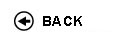Politecnico di Torino
Academic Year 2009/10
01LQRDR, 01LQRAX, 01LQRJA
Probability and statistics
1st degree and Bachelor-level of the Bologna process in Mechanical Engineering - Vercelli
1st degree and Bachelor-level of the Bologna process in Civil Engineering - Vercelli
1st degree and Bachelor-level of the Bologna process in Electronic And Computer Engineering - Vercelli
 Teacher Status SSD Les Ex Lab Tut Years teaching Puppo Gabriella30 20 0 0 3
 SSD CFU Activities Area context MAT/06 5 A - Di base Matematica, informatica e statistica
 Objectives of the course The course provides basic knowledge on statistics and probability theory. Expected skills Ability to solve applied problems involving basic probability theory and to analyze data with simple tools from statistics. Prerequisites Calculus I and II. Syllabus Probability. Introduction to probability and counting. Axioms of probability. Conditional probability and Bayes theorem. Discrete random variables: binomial and Poisson distributions. Expectation and distribution parameters. Continuous random variables: Gamma and Normal distributions. Joint distributions: covariance and correlation, linear regression. Statistics. Point estimation. Central limit theorem. Statistical intervals based on a single sample. Inferences on the mean and on variance. Testing hypotheses. Laboratories and/or exercises Problem sessions will widen and deepen the topics treated in the lectures. Time will be also devoted to problem solving and working out examples and exercises. Bibliography Main text: J.S. Milton, J.C. Arnold Introduction to Probability and Statistics: Principles and Applications for Engineering and the Computing Sciences, 4th edition Mc Graw Hill international editions Problem solving and exercises: J.S. Milton, J.C. Arnold Student Solutions Manual to accompany Introduction to Probability and Statistics, 4th Edition Mc Graw Hill international editions Revisions / Exam The exam will consist in a test requiring the solution of three or four exercises and in an oral discussion on the solution strategy used in the test. The written test will account for roughly 80% of the final grade. Programma definitivo per l'A.A.2009/10© Politecnico di Torino
Corso Duca degli Abruzzi, 24 - 10129 Torino, ITALY